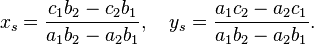## Intersection of Two Lines Calculator

 X +  Y = X +  Y =
 X Coordinate : Y Coordinate :

For the determination of the intersection point of two non-parallel lines

a1x+b1y=c1, a2x+b2y=c2

one gets from Cramer's rule for the coordinates of the intersection point (xs,ys)For instance: Line 1: y = 2x + 3, Line 2: y = -0.5x + 7

We start 2x + 3 = -0.5x + 7, Add 0.5x to each side 2.5x + 3 = 7, then x = 1.6

Place x = 1.6 into either of the equations then y = 6.2.

Thinkcalculator.com provides you helpful and handy calculator resources.